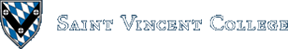Computing & Information Systems    DepartmentSearch CIS Site      Tutorials

## Software Design Using C++

### Pointers

We have used pointers informally throughout these Web pages and have pictured them as arrows. A pointer is probably implemented as the main memory address (or some variation on this) of the item to which it points. Pointers have been used to implement reference parameters on the run-time stack, arrays names were seen as pointers, and the this pointer was used with objects, just to name three uses. In addition we will later use pointers to construct dynamic data structures such as linked lists, stacks, queues, and binary trees.

A pointer variable is normally a pointer to a particular type of item. For example, we could have `p` be a pointer to an `int`. This would be set up as follows:

 `````` int * p; ``````

In the above, the `*` means the item that `p` points to. Thus it is saying that the item that `p` points to is an `int`. Of course, it is often more convenient to use a `typedef` to create a type name for any "pointer to an `int`".

### A Simple Example

Let's look at a simple example, pointers.cpp, to see how we might use pointers. Notice that a `typedef` has been set up to give the type name `IntPtrType`. `NumPtr` is then declared to have this type. `AgeOfMary` and `AgeOfBill` are set up as `int` variables. The code then does the following:

 `````` AgeOfMary = 19; AgeOfBill = 21; NumPtr = &AgeOfMary; // get address of the AgeOfMary variable cout << "After setting NumPtr to point to AgeOfMary, NumPtr is " << NumPtr << endl; cout << "NumPtr points to " << *NumPtr << endl; cout << "AgeOfMary is " << AgeOfMary << endl << "AgeOfBill is " << AgeOfBill << endl << endl; ``````

Note that in this context, the ampersand does not indicate a reference parameter, just a reference, a pointer to something, an address of something. You can think of it as the "address of" the item that follows. Also, remember that the `*` indicates the item pointed to by the variable that follows. The output from the above section of code might be something like that shown below. Note that the address contained in `NumPtr` can vary from computer to computer and even from one run of the program to another.

```After setting NumPtr to point to AgeOfMary, NumPtr is 0x0064FDF0
NumPtr points to 19
AgeOfMary is 19
AgeOfBill is 21
```

We might picture the above situation as follows.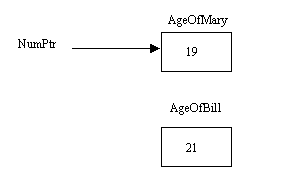The next section of code moves `NumPtr` so that it points to the `AgeOfBill` variable. The code is shown below.

 `````` NumPtr = &AgeOfBill; cout << "After moving NumPtr to point to AgeOfBill, NumPtr is " << NumPtr << endl; cout << "NumPtr points to " << *NumPtr << endl; cout << "AgeOfMary is " << AgeOfMary << endl << "AgeOfBill is " << AgeOfBill << endl << endl; ``````

The output for this section of code looks like this. Again, the exact address contained in the pointer may be different when you run the program.

```After moving NumPtr to point to AgeOfBill, NumPtr is 0x0064FDEC
NumPtr points to 21
AgeOfMary is 19
AgeOfBill is 21
```

The modified picture for the above situation is as follows: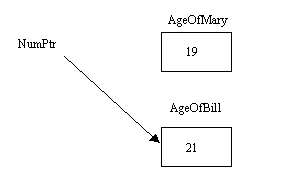As shown below, the next section of code changes `*NumPtr`, the item that the pointer currently points to. Thus, in this case we are changing what is in the variable `AgeOfBill`. Note that it is important to distinguish a pointer from the item to which it points.

 `````` *NumPtr = 22; cout << "After changing *NumPtr, NumPtr is " << NumPtr << endl; cout << "NumPtr points to " << *NumPtr << endl; cout << "AgeOfMary is " << AgeOfMary << endl << "AgeOfBill is " << AgeOfBill << endl << endl; ``````

Here is the output for the current section of code. Note that we can access the `22` via the pointer `NumPtr` or by looking in the `AgeOfBill` variable.

```After changing *NumPtr, NumPtr is 0x0064FDEC
NumPtr points to 22
AgeOfMary is 19
AgeOfBill is 22
```

The picture for the above is the same as before, except that you can see that a 22 has replaced the 21: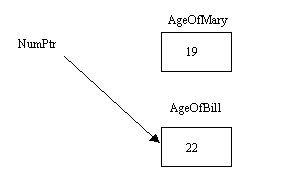Finally, we execute the last section of code, which is shown below. It changes our pointer variable so that it contains the special value `NULL`, which does not point at anything. Thus you cannot follow the pointer to try to examine the item pointed to, as is mentioned in the code itself.

 `````` NumPtr = NULL; cout << "After changing NumPtr to NULL, NumPtr is " << NumPtr << endl; cout << "We cannot print *NumPtr as NumPtr is NULL" << endl; cout << "AgeOfMary is " << AgeOfMary << endl << "AgeOfBill is " << AgeOfBill << endl << endl; ``````

The output from the above section is:

```After changing NumPtr to NULL, NumPtr is 0x00000000
We cannot print *NumPtr as NumPtr is NULL
AgeOfMary is 19
AgeOfBill is 22
```

The following picture shows the above situation: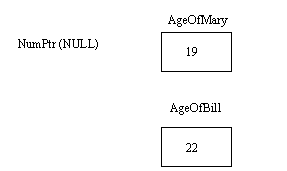### Using Pointers to Traverse Arrays

A pointer variable can be used to traverse the items in an array, as is shown in arrayptr.cpp. It is said that this is usually faster than using array indexing.

This example begins by setting up a pointer variable and an array. The array is initialized to contain some integer values. This section of code is approximately as shown below.

 `````` IntPtrType DataPtr; int A[MAX] = {100, 90, 80, 70, 60, 50, 40, 30, 20, 10}; ``````

Here is one way to write a loop to go through all of the items in the array, printing each item.

 `````` for (DataPtr = A; DataPtr < A + MAX; DataPtr++) cout << *DataPtr << endl; ``````

`DataPtr` is initially given a copy of the value of `A`. This might seem strange unless you remember that an array name is seen as a pointer to the first data item in the array. Thus, the value of `A` is `&A`, the address of `A`.

Also note that `A + MAX - 1` would be a pointer to the last item in the array. Pointer arithmetic is smart enough to take into account the size of the data items in the array. So, whether we have an array of integers or an array of rather large records, `A + 1` always points to `A`, `A + 2` points to `A`, etc.

We now see that the test in the above `for` loop makes sure that we do not run off of the end of the array. Note that one pointer is less than another provided that the first points to something that comes before (in memory) what the second points to.

The update step in the above loop simply adds 1 to the pointer, causing it to point to the next item in the array. Of course, the loop body prints out `*DataPtr`, the item pointed to by `DataPtr`.

The following is a picture of the array and associated pointers, with `DataPtr` shown at the point where it is pointing to `A`. Note that `A + MAX` points off the end of the array.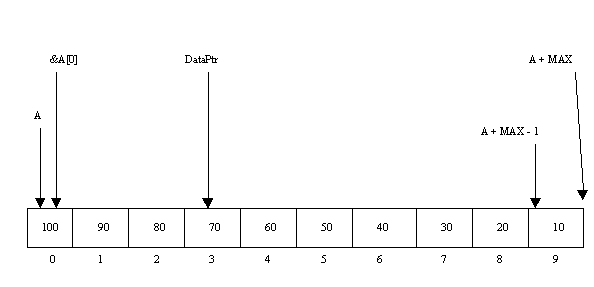### Dynamic Memory Allocation

Pointers also come into play when we want to allocate a chunk of memory while our program is running. This will be used later with various dynamic data structures. As a first example, let's look at dynamic.cpp, which begins by showing how to allocate space for an integer. The section of code which does this is as follows:

 `````` try { IntPtr = new int; } catch (bad_alloc) { cerr << "Could not allocate sufficient space" << endl; exit(1); } ``````

The `new` operation allocates space in the free store (often called the heap), which is a special region in the main memory area dedicated to your program. The `new` operation assigns the amount of space needed by the type of variable mentioned after it, in this case an `int`. If the `new` succeeds, it returns a pointer to the allocated space. If it fails, an exception of type `bad_alloc` is thrown. (See C++ Exceptions for more on exceptions.)

There is an older way to tell if the `new` operation to allocate memory has failed. In this scheme the `new` operation returns a `NULL` pointer. There is a way to get this older behavior if you prefer to use it instead of exception handling. The returning of a `NULL` pointer when the `new` operation fails can be turned on by using `new (nothrow)` like this:

 `````` IntPtr = new (nothrow) int; // Do not throw a bad_alloc exception if new fails. if (IntPtr == NULL) // Instead, return a NULL pointer. { cerr << "Could not allocate sufficient space" << endl; exit(2); } ``````

Your compiler settings may also affect which way `new` reports a memory allocation problem. Either way, once you have successfully allocated the chunk of memory, one then uses `*IntPtr` anytime access is needed to the space pointed to. That is, `*IntPtr` is the item pointed to by `IntPtr`. For example, our program places a number in this space and then prints it as follows:

 `````` *IntPtr = 44; cout << "The int pointed to by IntPtr is " << *IntPtr << endl << endl; ``````

When one is finished with dynamically allocated space, one should return it to the free store by using the `delete` operation as shown below. This allows the space to later be reused for something else. Although dynamically allocated space should ideally be freed up when your program ends, this does not always happen. A program that doesn't return all of the space that it allocates is said to suffer from memory leaks. After running the program one has less available memory than before, at least until the computer is restarted. Always reclaim any dynamically allocated space. In our example, this is done as follows:

 `````` delete IntPtr; ``````

The next section shows how to dynamically allocate space for an array of integers. The number of integers the array can hold is specified by the variable `NumItems`. Note that the user is asked to input the value for this variable. Thus, with dynamic memory allocation the user can input the number of items to use in the array at run-time, rather than having to specify a hard-coded number of items for the array before the program is even compiled. This is a key advantage of a dynamically-allocated array over a normal array variable. The actual allocation proceeds as follows:

 `````` try { IntPtr = new int[NumItems]; } catch (bad_alloc) { cerr << "Could not allocate sufficient space" << endl; exit(3); } ``````

Our example program also shows that you can check to see if the allocation failed by looking for a `NULL` pointer in the return value. Always check to see if a memory allocation failed and take appropriate action. If you fail to do so, your program may not work properly at times and could have security vulnerabilites as a result. Here is the version that checks for a `NULL` pointer to tell if the memory allocation worked or not:

 `````` IntPtr = new (nothrow) int[NumItems]; if (IntPtr == NULL) { cerr << "Could not allocate sufficient space" << endl; exit(4); } ``````

Once the requested memory has been successfully allocated, you can then use `IntPtr` as if it were an array, because for all practical purposes it is! The main difference is that an ordinary array is kept in a different place (in a stack frame on the run-time stack) in main memory than a dynamically allocated array (which is stored in the heap). Remember that an array name is seen as a pointer to the first data item, and that is precisely what `IntPtr` is. Thus you see the program accessing `IntPtr[k]` to get at the item at index `k` in the array. Here is that section of code:

 `````` for (k = 0; k < NumItems; k++) { cout << "Enter an integer to store: "; cin >> IntPtr[k]; } cout << endl << "The values stored in the array are:" << endl; for (k = 0; k < NumItems; k++) cout << IntPtr[k] << " "; ``````

One again uses the `delete` operation to free up space when finished with the allocated memory. However, with an array the `[]` brackets are given between the `delete` and the pointer variable as shown below:

 `````` delete [] IntPtr; ``````

Next, our program shows how to use pointers as strings. In one method, we set up pointer variable Msg1 as a pointer to a character, initialized as shown below. Space is allocated for a character array to hold `Section 5` since that is how the compiler handles literal strings such as this. The assignment statement merely copies the pointer to the first character (the 'S') into the `Msg1` variable. Note that the characters of the string do NOT get copied! One can then use something like `cout << Msg1;` to output the string.

 `````` typedef char * CharPtrType; CharPtrType Msg1 = "Section 5"; cout << Msg1; ``````

The other way shown for setting up a pointer as a string dynamically allocates space for the string. We use the `new` operator to do this, with either the scheme that throws an exception if the allocation fails or the version that returns a `NULL` pointer if sufficient space cannot be found. The program example shows both versions, but we only discuss the first one here.

`Msg2` is a pointer variable just like `Msg1`. Note the use of `strcpy` to copy the characters of the string into the newly allocated space. Note well that `strcpy` does not check to see if the destination string has enough room for the data. This can open up one's software to a buffer overflow attack, a well-known type of security flaw. When writing professional software use a copy function that does proper bounds checking instead. Follow the above link for further information on this important topic.

 `````` try { Msg2 = new char; } catch (bad_alloc) { cerr << "Could not allocate sufficient space" << endl; exit(6); } strcpy(Msg2, "Section 6"); cout << Msg2 << endl << endl; delete [] Msg2; // free up the space ``````

Overall, what is the main difference between the 2 methods, that used with Msg1 and that used with Msg2? Both used a literal string (characters between double quotes). However, with Msg1 we just had the pointer point to the existing literal string. With Msg2 we created a copy of the entire string of data. The second method would seem to be more wasteful, but there are times when a copy of the original string is exactly what we want.

Our example program ends with two versions of an interesting case of memory allocation. One version is the type that throws an exception if sufficient memory cannot be found, the other is the type that returns a `NULL` pointer if the new fails. We look just at the first of these two versions here:

 `````` try { Msg2 = new char[0x7fffffff]; // In Linux you may have to add 1 or 2 more letters f to get the error message. } catch (bad_alloc) { cerr << "Could not allocate sufficient space" << endl; exit(8); } strcpy(Msg2, "Section 8"); cout << Msg2 << endl << endl; delete [] Msg2; // free up the space ``````

Here we try to allocate a huge amount of memory space, an array of `0x7fffffff` characters. The `0x7fffffff` is a hexadecimal number (base 16, which uses digits 0 through 9 and a through f to represent 10 through 15). `7fffffff` would have decimal value 7 * (16 ^ 7) + 15 * (16 ^ 6) + 15 * (16 ^ 5) + 15 * (16 ^ 4) + 15 * (16 ^ 3) + 15 * (16 ^ 2) + 15 * (16 ^ 1) + 15 = 2,147,483,647. If each character is a simple ASCII character (not unicode), it takes one byte of storage, so that our code is asking for about 2 gigabytes of memory! That's probably enough in Windows to cause `new` to fail so that the "Could not allocate sufficient space" message gets displayed. This is a good test to see if your code is written correctly to detect these memory allocation failures.

### Dynamically Allocating Structures

Dynamically allocating a structure (struct) is similar to dynamically allocating simple data items like integers, floats, etc. We will use a PtrsToStructs.cpp example to illustrate how to use a pointer to dynamically allocate a structure, how to use that pointer to access the fields in the structure, and other related items.

First, this example sets up two types: `EmployeeType` is a type for a structure containing `Name`, `IDNum`, and `WageRate` fields. Then `EmployeePtrType` is for something that points to (dereferences to) an `EmployeeType` structure. Note the use of the asterisk as the dereferencing operator.

 `````` struct EmployeeType { string Name; int IDNum; float WageRate; }; typedef EmployeeType * EmployeePtrType; ``````

Two ways are shown in this example for using an `EmployeePtrType` pointer to dynamically allocate an `EmployeeType` structure. Let's look at the simpler one first.

The use of `new` to allocate space for a new structure and return a pointer to the allocated item is pretty much what we used above when allocating space for an `int` or `float`. As shown below, to access a field of a structure, we use an arrow of the form `->` between the pointer and the field. This is a shorthand notation in that `EmployeePtr->Name` really means `(*EmployeePtr).Name`, where we get the structure pointed at by applying the * dereferencing operator and then use the dot to pick out a particular field. The parentheses are needed to get the correct order of operations. Because that notation is clumsy, everyone uses the arrow notation.

The code below uses the arrow notation in two places. First, it uses this notation when we copy some data into the three fields of our dynamically allocated structure. Second, it again uses this notation to print out the data that is in this structure which our `EmployeePtr` points to.

 `````` EmployeePtrType EmployeePtr; EmployeePtr = new EmployeeType; EmployeePtr->Name = "Sally Keffer"; EmployeePtr->WageRate = 22.95; EmployeePtr->IDNum = 6438; cout << "Information on Employee:" << endl << endl; cout << "ID number: " << EmployeePtr->IDNum << endl; cout << "Name: " << EmployeePtr->Name << endl; cout << "Wage rate: " << EmployeePtr->WageRate << endl << endl; delete EmployeePtr; // Don't forget to recover the allocated space when you are done with it. ``````

A better way to dynamically allocate the structure is also shown in our example. It places the use of `new` to dynamically allocate a structure inside the `try` portion of a `try...catch` construct. If any exception is raised by the code in the `try` part, the `catch` portion will print the error message shown below. The ... after the `catch` indicates that all types of errors should be caught here. The most likely error is the `bad_alloc`, discussed above, that occurs when the `new` operation fails to allocate space for the requested item. Simply catching exceptions of type `bad_alloc` would be sufficient. Still, if we had additional lines of code in the try section and used ... to catch any exception, then if an exception were raised by any line of code in the try portion, it would be caught and the error message would be printed.

 `````` EmployeePtrType p; try { p = new EmployeeType; } catch (...) { cerr << "Could not allocate memory for a new EmployeeType structure." << endl << endl; exit(1); } p->Name = "Sam Snead"; p->WageRate = 20.45; p->IDNum = 1207; cout << "Information on Employee:" << endl << endl; cout << "ID number: " << p->IDNum << endl; cout << "Name: " << p->Name << endl; cout << "Wage rate: " << p->WageRate << endl << endl; delete p; // Don't forget to recover the allocated space when you are done with it. ``````

### Dynamically Allocating Objects

#### First Example

The dyobject.cpp example shows how to dynamically allocate and delete an object. Allocating an object dynamically works essentially the same as dynamically allocating a structure. Here we use the class of `Product` objects that is declared and defined in product.h and product.cpp.

The code to create, print, and remove such a `Product` object is shown below. Note that we again use a pointer to the dynamically created item. The `new` operation uses the constructor function to initialize the data in the object. In this example the constructor takes three parameters: the name of the product, the year it was manufactured, and the price.

 `````` Product StaticProd("Sample product (static)", 1998, 14.95); ProductPtrType DynamicProdPtr; DynamicProdPtr = new Product("Another product (dynamic)", 1997, 9.33); // If the new fails, a bad_alloc exception is raised. // Since that exception is unhandled, the program would abort. // Next, let's use the two objects: cout << "Printing the static object:" << endl; StaticProd.Print(); cout << "Printing the dynamic object:" << endl; DynamicProdPtr->Print(); cout << "Printing the dynamic object in another way:" << endl; (*DynamicProdPtr).Print(); // Delete space used by the dynamic object -- uses the destructor: delete DynamicProdPtr; ``````

In this example, we chose not to handle the bad_alloc exception that is typically raised if `new` fails to allocate the requested memory space. Thus our program would abort if a bad_alloc exception happened. You might want to look at the previous example on this page and at the C++ Exceptions section of these web pages to see if you can modify this example to handle the bad_alloc exception.

Note the use above of the `->` to access a class function of the object pointed to by `DynamicProdPtr`. This is really just a convenient shortcut for the clumsier notation `(*DynamicProdPtr).Print()`. It is clear from the latter that we find the object pointed to and then call its `Print` function. (The same arrow notation is also used to pick out a field of a structure when given a pointer to the structure.)

Finally, note that when the `delete` operation is used on the pointer to an object, the destructor class function is called. An automatic destructor is always provided which reclaims the space for the object itself, but sometimes you need to write your own. This is the case with our `Product` objects, because the name string itself is not stored within the object. Instead, each object contains a pointer to the name string, which is external to the object. This was done deliberately to show how to handle such a case. Using the default destructor would reclaim the space for the object itself, but would not free up the space used by this external string.

The answer to this problem is to write one's own destructor that explicitly frees up the space used by this string. A destructor function always uses as its function name the name of the class preceded by a `~` symbol. Note the entry in the class declaration for this `~Product` function. The actual code for the function is pasted in below for convenience.

 `````` Product::~Product(void) { // Print a message just so that you can see when the destructor // gets called: cout << "Destructor called for product named " << NamePtr << endl; // Explicitly reclaim the space used by the string: delete [] NamePtr; } ``````

Normally a destructor would not print a message. This is done here just so that when you run the program you will be able to see when the destructor gets called. Try it! You will see that besides getting called for the dynamically allocated object, the destructor gets called when an ordinary "static" object goes out of scope (in this case because the program ends).

#### Second Example

This example shows two ways of creating and using an array of objects, as well as a related method of using an array of pointers to objects. The complete code is found in product2.h, product2.cpp, and example2.cpp.

In this example, we use a modified version of the Product class. This new version uses a string class object, instead of a character array string, for the Name field. The Price field has also been changed, in this case to be a double instead of a float.

 `````` /* Filename: product2.h Author: Br. David Carlson Date: March 18, 2017 This file sets up the Product class and ProductPtrType. Note that the class Product uses a string object for the Name field. */ #include #include using namespace std; class Product { private: string Name; int Year; double Price; public: Product(string InitialName = "NO_NAME_GIVEN", int InitialYear = 0, double InitialPrice = 0); ~Product(void); void Print(void) const; void SetName(string NameValue); void SetYear(int YearValue); void SetPrice(double PriceValue); string GetName(void) const; int GetYear(void) const; double GetPrice(void) const; }; typedef Product * ProductPtrType; ``````

The product2.cpp is very similar to the old product.cpp file. The main difference is that assignment can be used with string objects. The use of the dangerous strcpy function is eliminated. You see this, for example, in the SetName method as shown below.

 `````` /* Given: NameValue A string. Task: To place NameValue into the Name field of the implicit object. Return: Nothing directly, though the implicit object is updated. */ void Product::SetName(string NameValue) { Name = NameValue; } ``````

The following section of code shows the constant and types that are set up in example2.cpp:

 `````` const int MAX = 3; typedef Product ProductArrayType[MAX]; typedef ProductPtrType ProductPtrArrayType[MAX]; ``````

Then the statically created array is set up and used as follows. See example2.cpp to see this code in context.

 `````` cout << endl << "Start of function TestStaticArrayObjects, before declaring the array of objects" << endl; ProductArrayType ProductArray; // This is our array of objects (with default values to start with) string NameTemp, Junk; int YearTemp; double PriceTemp; // We ask the user for the data to put into each of the objects in ProductArray. cout << endl << "You will now be asked to enter data for the array of objects." << endl; for (int k = 0; k < MAX; k++) { cout << "Enter the name for product " << k << " : "; getline(cin, NameTemp); cout << "Enter the year for product " << k << " : "; cin >> YearTemp; cout << "Enter the price for product " << k << " : "; cin >> PriceTemp; getline(cin, Junk); // Clear out the input buffer. ProductArray[k].SetName(NameTemp); ProductArray[k].SetYear(YearTemp); ProductArray[k].SetPrice(PriceTemp); } // Now we print the data. cout << endl << "Here is the data that your entered:" << endl; for (int k = 0; k < MAX; k++) { ProductArray[k].Print(); } cout << "End of function TestStaticArrayObjects" << endl; ``````

When example2.cpp is run, the first section of output is largely due to the section of code above for the statically created array of objects. Note that the Product class constructor got called three times when an array of three Product objects was statically created (by simply declaring it). Since the code above is inside of a function named TestStaticArrayObjects, when the end of that function is reached, the array of objects goes out of scope. Thus the destructor is automatically called on each of the three objects in the array.

 ```Start of main function Start of function TestStaticArrayObjects, before declaring the array of objects Constructor called for object with name NO_NAME_GIVEN Constructor called for object with name NO_NAME_GIVEN Constructor called for object with name NO_NAME_GIVEN You will now be asked to enter data for the array of objects. Enter the name for product 0 : A Enter the year for product 0 : 2000 Enter the price for product 0 : 1234.56 Enter the name for product 1 : B Enter the year for product 1 : 2001 Enter the price for product 1 : 2345.67 Enter the name for product 2 : C Enter the year for product 2 : 2002 Enter the price for product 2 : 3456.78 Here is the data that your entered: Product name: A Year made: 2000 Price: 1234.56 Product name: B Year made: 2001 Price: 2345.67 Product name: C Year made: 2002 Price: 3456.78 End of function TestStaticArrayObjects Destructor called for product named C Destructor called for product named B Destructor called for product named A ```

The next section of code in example2.cpp deals with the dynamically allocated array of Product objects. Note that the user is asked how many products are to be put into the array. Then the array is allocated so as to be of just the right length.

 `````` cout << endl << "Start of function TestDynamicArrayObjects, before allocating the array of objects" << endl; // Dynamic Array is a pointer to the start of the array, but it is also a name for the array. ProductPtrType DynamicArray; int NumProducts; string NameTemp, Junk; int YearTemp; double PriceTemp; cout << "How many products do you want to put in the dynamically allocated array? "; cin >> NumProducts; getline(cin, Junk); // Clear out the input buffer. try { DynamicArray = new Product[NumProducts]; } catch (bad_alloc) { cerr << "Could not allocate sufficient space" << endl; exit(1); } for (int k = 0; k < NumProducts; k++) { cout << "Enter the name for product " << k << " : "; getline(cin, NameTemp); cout << "Enter the year for product " << k << " : "; cin >> YearTemp; cout << "Enter the price for product " << k << " : "; cin >> PriceTemp; getline(cin, Junk); // Clear out the input buffer. DynamicArray[k].SetName(NameTemp); DynamicArray[k].SetYear(YearTemp); DynamicArray[k].SetPrice(PriceTemp); } // Now we print the data. cout << endl << "Here is the data that your entered:" << endl; for (int k = 0; k < NumProducts; k++) { DynamicArray[k].Print(); } // You must call delete to reclaim the space for the array of objects: delete [] DynamicArray; cout << "End of function TestDynamicArrayObjects" << endl; ``````

The following is a sample interaction with this portion of the program. You can see that the user asks for a two-item array, and that the default constructor puts NO_NAME_GIVEN in as the name for both Product objects. The user then enters data, which is then put into the two objects using the set functions. The Print function then shows what is in the two objects. Note that when delete is called on DynamicArray, not only is the array deleted, but also the destructor gets run on both of the objects in the array.

 ```Start of function TestDynamicArrayObjects, before allocating the array of objects How many products do you want to put in the dynamically allocated array? 2 Constructor called for object with name NO_NAME_GIVEN Constructor called for object with name NO_NAME_GIVEN Enter the name for product 0 : D Enter the year for product 0 : 2005 Enter the price for product 0 : 4567.89 Enter the name for product 1 : E Enter the year for product 1 : 2006 Enter the price for product 1 : 5678.90 Here is the data that your entered: Product name: D Year made: 2005 Price: 4567.89 Product name: E Year made: 2006 Price: 5678.90 Destructor called for product named E Destructor called for product named D End of function TestDynamicArrayObjects ```

In the last section of example2.cpp, a statically created array of pointers is used. Each pointer points to a dynamically allocated Product object. Technically, we do not have an array of objects here, but our array of pointers is closely-related.

 `````` cout << endl << "Start of function TestArrayOfObjectPointers, before declaring the array of pointers to objects" << endl; ProductPtrArrayType ProductPtrArray; // This is our array of pointers to objects. string NameTemp, Junk; int YearTemp; double PriceTemp; // We ask the user for the data to put into each of the objects pointed to in ProductPtrArray. cout << endl << "You will now be asked to enter data for each object pointed to in ProductPtrArray." << endl; for (int k = 0; k < MAX; k++) { try { ProductPtrArray[k] = new Product; } catch (bad_alloc) { cerr << "Could not allocate sufficient space" << endl; exit(2); } cout << "Enter the name for product " << k << " : "; getline(cin, NameTemp); cout << "Enter the year for product " << k << " : "; cin >> YearTemp; cout << "Enter the price for product " << k << " : "; cin >> PriceTemp; getline(cin, Junk); // Clear out the input buffer. ProductPtrArray[k]->SetName(NameTemp); ProductPtrArray[k]->SetYear(YearTemp); ProductPtrArray[k]->SetPrice(PriceTemp); } // Now we print the data. cout << endl << "Here is the data that your entered:" << endl; for (int k = 0; k < MAX; k++) { ProductPtrArray[k]->Print(); } // You must call delete to reclaim the space for each object: for (int k = 0; k < MAX; k++) { delete ProductPtrArray[k]; } cout << "End of function TestArrayOfObjectPointers" << endl; ``````

Here is sample input and output when running this final section of the program. You can see where the constructor makes a new Product object with default values in it. Then the user is prompted for the data to put into the new object. At the end, when delete is done on each of the pointers in the array, that calls the destructor on the object that each pointer points to.

 ```Start of function TestArrayOfObjectPointers, before declaring the array of pointers to objects You will now be asked to enter data for each object pointed to in ProductPtrArray. Constructor called for object with name NO_NAME_GIVEN Enter the name for product 0 : F Enter the year for product 0 : 2010 Enter the price for product 0 : 4444.44 Constructor called for object with name NO_NAME_GIVEN Enter the name for product 1 : G Enter the year for product 1 : 2011 Enter the price for product 1 : 6666.66 Constructor called for object with name NO_NAME_GIVEN Enter the name for product 2 : H Enter the year for product 2 : 2012 Enter the price for product 2 : 8888.88 Here is the data that your entered: Product name: F Year made: 2010 Price: 4444.44 Product name: G Year made: 2011 Price: 6666.66 Product name: H Year made: 2012 Price: 8888.88 Destructor called for product named F Destructor called for product named G Destructor called for product named H End of function TestArrayOfObjectPointers End of main function ```